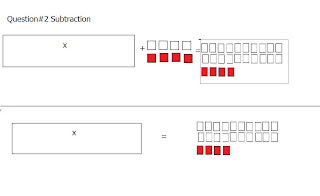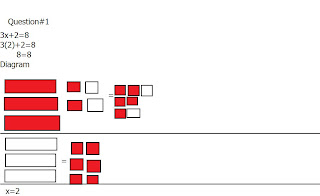## Monday, May 9, 2011

### Paulo's Algebra Post

One Step Algebra Equation:

Variable: is a letter that represents an unknown number in algebra.( q, x, z, t)

Constant
: is the integer in an expression or equation.( x +6 )

Expression
: is a pattern.( 2x )

Equation
:has an equal sign. only one possible answer.( 2x=6)

-Isolate the variable
-Cancel using zero pair for the constant
-Balance on the other side of the equal signSubtractionSolving Addition/Subtraction One Step Equations Using Algebraic Tiles:
Isolate the variable
Cancel the constant using a zero pair
Balance
Verify to checkSubtractionSolving Multiplication/Dividing One Step Equations:
Isolate the variable
Cancel using the opposite
multiply or divide
Balance
Verify

Solving Multiplication/Dividing One Step Equations Using Algebraic Tiles:
Isolate the variable
Simplify the variable

Balance
Verify

MultiplicationDivision